×#### Thank you for registering.

One of our academic counsellors will contact you within 1 working day.

Click to Chat

1800-1023-196

+91-120-4616500

CART 0

• 0

MY CART (5)

Use Coupon: CART20 and get 20% off on all online Study Material

ITEM
DETAILS
MRP
DISCOUNT
FINAL PRICE
Total Price: Rs.

There are no items in this cart.
Continue Shopping• Complete JEE Main/Advanced Course and Test Series
• OFFERED PRICE: Rs. 15,900
• View Details

3D Geometry

The cosines of the angle made by a line with coordinate axes are called the direction cosines of that line. In the adjoining figure, let α, ß, γ be the angles made by a line AB with coordinate axes then cos α, cos ß, cos γ are the direction cosines of AB which are generally denoted by l, m, n.Hence, l = cos α, m = cos ß, n = cos γ

DCs ∈ [-1, 1].

Direction cosines of the coordinate axis:

dc’s of x-axis = 1, 0, 0.

dc’s of y-axis = 0, 1, 0.

dc’s of z-axis = 0, 0, 1.

The direction cosines of a line parallel to any coordinate axis are equal to the direction cosines of the corresponding axis.

l2 + m2 + n2 = 1.

If a, b, c are such numbers which are proportional to the direction cosines l, m, n of a line then a, b, c are direction ratios of the line.

a, b, c are drs ⇔ a/l = b/m = c/n

Number of drs is not unique where as number of dcs is unique.

a2 + b2 + c2 ≠ 1.

cos α = ± a/√(a2 + b2 + c2)

cos β = ± b/√(a2 + b2 + c2)

cos γ = ± c/√(a2 + b2 + c2)

The direction ratios of the line segment joining P(x1, y1, z1) and Q(x2, y2, z2) may be taken as x2 - x1, y2 - y1, z2 - z1 or x1 - x2, y1 - y2, z1 - z2.

The direction cosines of the line segment joining P(x1, y1, z1) and Q(x2, y2, z2) may be taken as

, ,

Angle Between two lines:

?Case 1: When dcs of the lines are given:

Let us assume that θ is the angle between the two lines say AB and AC whose direction cosines are l1, m1 and n1 and l2, m2 and n2 then?

cos θ = l1l2 + m1m2 + n1n2

?Case 2: When drs of the lines are given:

If the direction ratios of two lines a1, b1, c1 and a2, b2 and c2 then the angle between two lines is given by

If the direction cosines of a line segment AB are (l, m, n) then those of line BA will be (-l, -m, -n).

Conditions for two lines to be parallel or perpendicular:

If two lines are parallel then the angle between them is 0° and if they are perpendicular then the angle is 90°. Hence, we have the following cases:

?Case 1: When dcs of the lines AB and CD, say l1, m1 and n1 and l2, m2 and n2 are given:

?AB || CD ⇔ l1 = l2 , m1 = m2 and n1 = n2

AB ⊥ CD ⇔ l1l2 + m1m2 + n1n2 = 0

?Case 2: When drs of the lines AB and CD, say a1, b1 and c1 and a2, b2 and c2 are given:

?AB || CD ⇔ a1/a2 = b1/b2 = c1/c2

AB ⊥ CD ⇔ a1a2 + b1b2 + c1c2 = 0.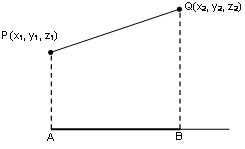??Let PQ be a line segment where P = (x1, y1, z1) and Q = (x2, y2, z2) and AB be a given line with dc’s as l, m, n. If the line segment PQ makes an angle θ with the line AB, then the projection of PQ is P’Q’ = PQ cos θ, hence

P’Q’ = l(x2 - x1) + m(y2 - y1) + n(z2 - z1)

We know that for x-axis, we have l = 1 and m = n = 0. Hence,

Projection of PQ on x- axis = 1(x2 - x1) + 0(y2 - y1) + 0(z2 - z1) = (x2 - x1)

Projection of PQ on z- axis = 0(x2 - x1) + 0(y2 - y1) + 1(z2 - z1) = (z2 - z1)

We know that PQ2 = (x2 - x1)2 + (y2 - y1)2 + (z2 - z1)2 which shows that if a, b, c are the projections of a line segment on coordinate axis then the length of the segment = √(a2 + b2+ c2)

If a, b, c are the projections of a line segment on coordinate axis then its dc’s are ± a/√(a2 + b2 + c2), ± b/√(a2+ b2 + c2), ± c/√(a2 + b2 + c2)

Perpendicular distance of a point from a line:

Let AB be the straight line passing through the point A(a, b, c) and having direction cosines l, m, and n. Now, if AN is assumed to be the projection of line AP on the straight line AB then we have

AN = l(x – a) + m(y – b) + n(z – c)

and AP = √(x–a)2 + (y–b)2 + (z–c)2

∴ Perpendicular distance of point P

PN = √(AP2 – AN2)

?    Equation of straight line passing through point P (x1, y1, z1) and whose direction cosines are l, m, n is

(x – x1)/l =  (y – y1)/m = (z – z1) / n.

Equation of straight line passing through two points P (x1, y1, z1) and Q (x2, y2, z2) is

(x – x1)/(x2 – x1) = (y – y1 )/(y2 – y1) = (z – z1)/(z2 – z1)

Foot, length and equation of perpendicular from a point to a line:

Let the equation of line be

and (α, ß, γ) be the point. Let l, m , n denote the actual dcs of the line. Then

Equation of perpendicular:

Equation of any plane through a given line:

Equation of any plane through the line

is A(x – x1) + B(y – y1) + C(z – z1) = 0, where Al + Bm + Cn = 0.

Let the two lines be ax + by + cz + d = 0 = a’x + b’y + c’z + d’ and αx + ßy + γz + δ = 0 = α’x + ß’y + γ’z + δ’. These are coplanar ifThe straight lines which are not parallel and non-coplanar i.e. non-intersecting are called skew-lines.

Let the equations of lines be

and

Then shortest distance between them is given by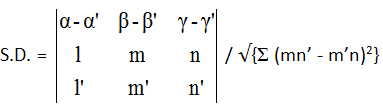If the lines intersect, the S.D. between them is zero. Therefore,General equation of a plane is ax + by + cz + d = 0.

Equation of the plane in Normal form is lx + my + nz = p where p is the length of the normal from the origin to the plane and (l, m, n) be the direction cosines of the normal.

The equation of the plane passing through P(x1, y1, z1) and having direction ratios (a, b, c) for its normal is a (x – x1) + b (y – y1) + c (z – z1) = 0

The equation of the plane passing through three non-collinear points (x1, y1, z1), (x2, y2, z2) and (x3, y3 , z3) is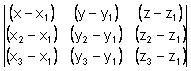= 0

The equation of the plane whose intercepts are a, b, c on the x, y, z axes respectively is x/a + y/b + z/c = 1.

Angle between the planes is defined as angle between normals of the planes drawn from any point to the planes.

Angle between the planes a1x + b1y + c1z + d1 = 0 and a2x + b2y + c2z + d2 = 0 is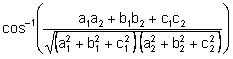If a1a2 + b1b2 + c1c2 = 0, then the planes are perpendicular to each other.

If a1/a2 = b1/b2 = c1/c2 then the planes are parallel to each other.

The length of the perpendicular from the point P(x1, y1, z1) to the plane ax + by + cz + d = 0 is |ax1 + by1 + cz1 + d| / √a2 + b2 + c2.

??Equation of plane passing through the line of intersection of two planes u = 0 and v = 0 is u + λv = 0.

Bisectors of angles between two planes:

The equation of the planes bisecting the angles between two given planes a1x +b1y +c1z +d1 = 0 and a2x + b2y + c2z +d2 = 0 is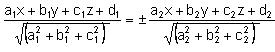If equation of a plane is ax + by + cz + d = 0, then direction cosines of normal to this plane are a, b, c. So angle between normal to the plane and a straight line having direction cosines l, m ,n is given by cos θ = al + bm + cn / √a2 + b2 + c2. Then angle between the plane and the straight line is π/2 – θ.

Plane and straight line will be parallel if al + bm + cn = 0

Plane and straight line will be perpendicular if a/l = b/m = c/n.

If S = o and S’= 0 intersect, then S – S’ = 0 represents the equation of common plane of intersection and this intersection yields a circle.?

r = √u2 + v2 + w2 - k is the radius of the sphere. Then,

p < r plane intersects the sphere

p = r plane touches the sphere

p > r plane neither intersects nor touches the sphere.

A line segment joining two points on a sphere and passing through the centre is called a diameter.

The shortest possible distance between any two points on a sphere is termed as geodesic.

The surface area of the sphere of radius ‘r’ is given by A = 4πr2

The volume of the sphere of radius ‘r’ is given by V = 4/3 πr3

If there are two spheres S = x2 + y2 + z2 + 2ux + 2vy + 2wz + d = 0 and S’ = x2 + y2 + z2 + 2u’x + 2v’y + 2w’z + d’ = 0. Here C(-u, -v, -w) and C’ = (-u’, -v’, -w’) are their centres and if r, r’ be their radii then:

?CC’ > r + r’ ⇒ spheres are exterior to each other i.e. neither intersecting nor including.

|r – r’| < CC’ < r + r’ Spheres are intersecting

CC’ = |r + r’| ⇒ The they touch each other externally

CC’ = |r – r’| ⇒The they touch each other internally

CC’ < |r – r’| ⇒ one is contained in the other

Let P = ax + by + cz + d = 0 be the plane and S = x2 + y2 + z2 + 2ux + 2vy + 2wz + k = 0 be a sphere. Let p be the length of perpendicular from the centre (-u, -v, -w) of sphere to the plane P = 0.

r = √(u2 + v2 + w2 - k) is the radius of the sphere. Then

p < r ⇒ plane intersects sphere

p = r ⇒ plane touches sphere

p > r ⇒ plane neither intersects nor touches the sphere### Course Features

• 728 Video Lectures
• Revision Notes
• Previous Year Papers
• Mind Map
• Study Planner
• NCERT Solutions
• Discussion Forum
• Test paper with Video Solution